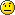## CULASYEVX Vs Matlab EIGS

General CULA Dense (LAPACK & BLAS) support and troubleshooting. Use this forum if you are having a general problem or have encountered a bug.

### CULASYEVX Vs Matlab EIGS

Im very very surprised that CULASYEVX is fully defeated by Matlab EIGS, its performance is very very far away from the EIGS, in fact we do some tests of different matrixs, from 500x500 to 12500x12500(double precision), the CULASYEVX consumed many many times from 47 to 365 times than the EIGS.eigs.jpg (25.04 KiB) Viewed 8297 times
Table : Performance of 5 maximums EigenValues and EigenVectors,time in Second. Tesla C1060 with CULA3.1

I wanna know if i get something wrong here or not?

Lab. TREFLE France.
asempey

Posts: 11
Joined: Tue Sep 21, 2010 1:52 am

### Re: CULASYEVX Vs Matlab EIGS

These are dense matrices being used in MATLAB?
kyle

Posts: 301
Joined: Fri Jun 12, 2009 7:47 pm

### Re: CULASYEVX Vs Matlab EIGS

kyle wrote:These are dense matrices being used in MATLAB?

yes, dense matrices
asempey

Posts: 11
Joined: Tue Sep 21, 2010 1:52 am

### Re: CULASYEVX Vs Matlab EIGS

At first glance, your runtimes for MATLAB's eigs() seems really low. On my machine (Core i7), my runtime is ~20x longer.

Code: Select all
`>> A = rand(12500,12500); A = A*A';>> tic; [V,E] = eigs(A); toc;Elapsed time is 41.908515 seconds.`

Also, I won't have the facilities to check right now, but the CULA runtime seems a bit high as well.
kyle

Posts: 301
Joined: Fri Jun 12, 2009 7:47 pm

### Re: CULASYEVX Vs Matlab EIGS

thx for ur reply, the matrix we are used to test is not a random,
here i give a snapshot of my matrix(not random)data.jpg (49.21 KiB) Viewed 8286 times
so this could be a reason why my runtime matlab is so low. However for a random matrix
i got the following:
Code: Select all
`>> tic; [V,E] = eigs(WC,5); toc;Elapsed time is 2.758782 seconds.>> A = rand(12500,12500); A = A*A';tic; [V,E] = eigs(A,5); toc;Elapsed time is 33.342538 seconds.`

the matlab eigs is high just like ur test. (intel i7 975 matlab 2010)

wish u find out the reason why cula is so high! CULASYEVX is really not a good idea when we need just some EigenValues and EigenVectors, it takes many many times.
asempey

Posts: 11
Joined: Tue Sep 21, 2010 1:52 am

### Re: CULASYEVX Vs Matlab EIGS

According to the MATLAB documentation, EIGS is implemented using the Implicitly Restarted Arnoldi Method. This is an iterative method whose runtime will greatly vary based on your matrix properties, or simply not solve some matrices.

In CULA, we have implemented our symmetric eigenvalue solver using a direct tridiagonalization + implicit QR/QL iteration method. This is what MATLAB's eig routine uses under the hood.

Fortunately, we are currently working on a iterative package that will include the IRA method for both full and sparse matrices.
kyle

Posts: 301
Joined: Fri Jun 12, 2009 7:47 pm

### Re: CULASYEVX Vs Matlab EIGS

Ok, got it, thx again kyle!
asempey

Posts: 11
Joined: Tue Sep 21, 2010 1:52 am